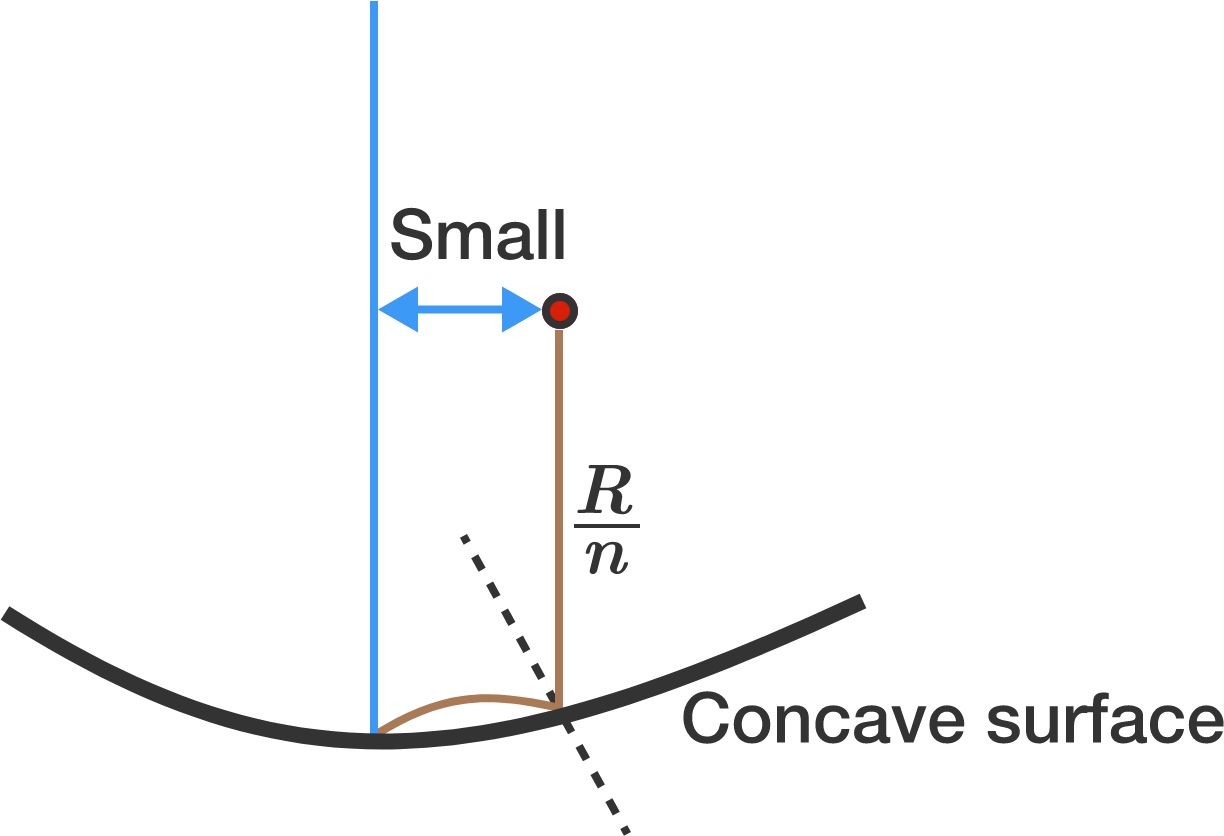# Balls bouncing on a concave surfaceA ball with zero initial velocity falls from a height of $\frac{R}{n}$ near the vertical axis of symmetry on a concave spherical surface of radius $R$. Assuming that the collision is elastic, it is observed that the second impact of the ball is at the lowest point of the spherical surface. Determine the value of $n$ to the nearest integer.

×